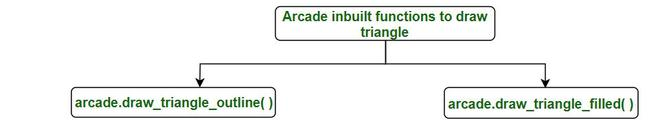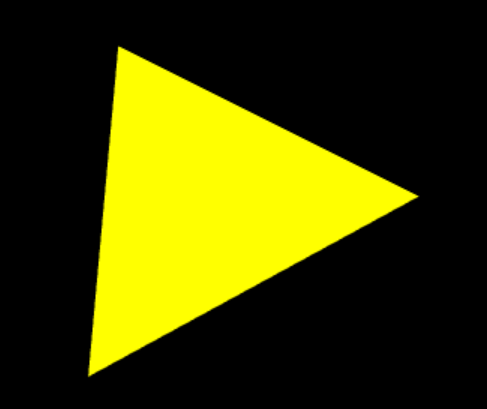Draw a triangle using Arcade in Python

• Difficulty Level : Expert
• Last Updated : 19 Oct, 2020

Arcade is a Python library that is used for developing 2Dimensional Games. Arcade needs support for OpenGL 3.3+. In the arcade, basic drawing does not require knowledge on how to define functions or classes or how to do loops, simply we have inbuilt functions for drawing primitives.

Arcade inbuilt function for drawing triangle:1: arcade.draw_traingle_outline( ): This function is used to draw an outline of a triangle.

Syntax: arcade.draw_triangle_outline(x1 , y1, x2 , y2 , x3 , y3 , color, border_width)

Parameters:

• x1: x value of first coordinate.
• y1: y value of first coordinate.
• x2:x value of second coordinate.
• y2: y value of second coordinate.
• x3: x value of third coordinate.
• y3: y value of third coordinate.
• color: Outline color of the triangle.
• border_width: Width of the border in pixels. Defaults to 1.

Let’s see an example to understand its functioning better.

Python3

Output:2: arcade.draw_triangle_filled( ): This function is used to draw a triangle filled with color.

Syntax: arcade.draw_triangle_filled(x1, y1, x2, y2 , x3 , y3, color)

Parameters:

• x1: x value of first coordinate.
• y1: y value of first coordinate.
• x2: x value of second coordinate.
• y2: y value of second coordinate.
• x3: x value of third coordinate.
• y3: y value of third coordinate.
• color: color to be filled in triangle.

Example:

Python3